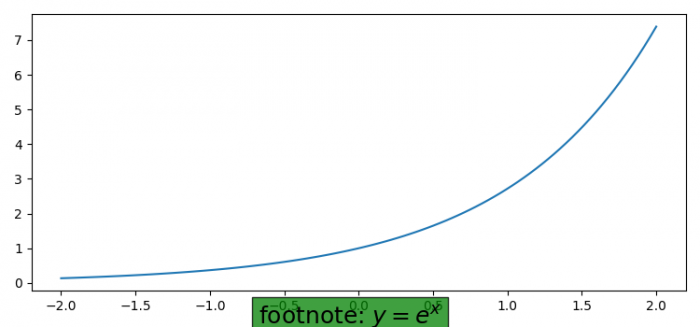# How to add footnote under the X-axis using Matplotlib?

To add footnote under the X-axis using matplotlib, we can use figtext() and text() method.

## Steps

• Set the figure size and adjust the padding between and around the subplots.
• Create x and y data points using numpy.
• Plot x and y data points using numpy.
• To place the footnote, use figtext() method with x, y position and box properties.
• To display the figure, use show() method.

## Example

import numpy as np
import matplotlib.pyplot as plt

plt.rcParams["figure.figsize"] = [7.50, 3.50]
plt.rcParams["figure.autolayout"] = True

x = np.linspace(-2, 2, 100)
y = np.exp(x)

plt.plot(x, y)
plt.figtext(0.5, 0.01, "footnote: $y=e^{x}$", ha="center", fontsize=18, bbox={"facecolor": "green", "alpha": 0.75, "pad": 5})
plt.show()

## Output